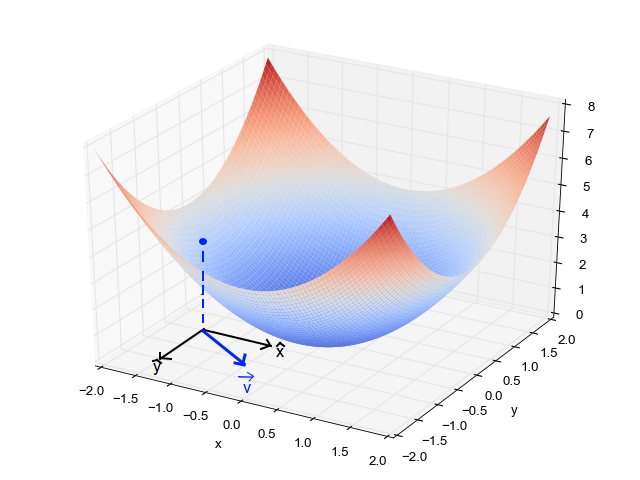# The Power of Gradient Descent: An In-depth Guide to the Pinnacle of Optimization Algorithms### Introduction

The world of machine learning and artificial intelligence (AI) is driven by algorithms that learn from data and improve their performance over time. At the heart of many of these algorithms, particularly in the field of supervised learning, lies a powerful optimization technique known as ‘Gradient Descent.’ This extensive guide dives deep into the workings of Gradient Descent, detailing its role in machine learning and the various forms it takes.

### The Essence of Machine Learning and Optimization

Before delving into Gradient Descent, let’s establish an understanding of machine learning’s core principles. Machine learning is a branch of AI where algorithms learn patterns from data, allowing them to make predictions or decisions without being explicitly programmed to perform the task.

The fundamental aspect of machine learning involves optimizing a function, often referred to as a cost or loss function. The goal is to find the set of parameters that minimizes this function, which represents the difference between the algorithm’s predictions and the actual values. This is where Gradient Descent comes into play.

Gradient Descent is a first-order optimization algorithm used to find the local minimum of a function. In the context of machine learning, it’s employed to minimize the cost function, consequently improving the model’s performance.

Conceptually, Gradient Descent can be thought of as a hiker (the algorithm) trying to find the lowest point in a valley (the minimum of the cost function) by taking steps in the direction of the steepest descent.

### The Mechanics of Gradient Descent

Gradient Descent operates iteratively, adjusting the model’s parameters step by step to reduce the cost function. The size of these steps is determined by the learning rate, a crucial hyperparameter in Gradient Descent.

A high learning rate might make large steps, allowing the algorithm to converge quickly, but it risks overshooting the minimum point. Conversely, a low learning rate results in smaller, more precise steps, but the algorithm may take longer to converge, or it may get stuck if the steps are too small.

While the core principle remains the same, Gradient Descent takes different forms depending on how much data is used to compute the gradient of the cost function:

Batch Gradient Descent: Uses the entire training dataset to compute the gradient of the cost function at each step. Although computationally intensive, it provides a stable path towards the minimum.

Stochastic Gradient Descent (SGD): Uses a single randomly-selected training example to compute the gradient at each step. While it’s faster and can escape local minima better than Batch Gradient Descent, SGD tends to have a much noisier convergence, bouncing around the minimum value.

Mini-Batch Gradient Descent: A compromise between Batch and Stochastic Gradient Descent. It uses a mini-batch of n training examples at each step. This variant reduces the noise in SGD while being more computationally efficient than Batch Gradient Descent.

### Challenges in Implementing Gradient Descent

Despite its efficiency, Gradient Descent isn’t without challenges. The choice of the learning rate is critical — too small, and the algorithm will be slow to converge; too large, and the algorithm might overshoot the minimum or diverge entirely. Furthermore, Gradient Descent’s performance can be affected by the shape and structure of the cost function. It may get stuck in local minima or saddle points, particularly in high-dimensional spaces.

Several enhancements, such as Momentum, RMSProp, and Adam, have been proposed to overcome these challenges, combining the concepts of Gradient Descent with sophisticated techniques to accelerate convergence and ensure a smoother path towards the minimum of the cost function.

### Conclusion

In the world of machine learning, Gradient Descent stands as a testament to the power of simple ideas. This optimization algorithm, with its iterative approach to minimizing cost functions, is a cornerstone of many machine learning models. Understanding its mechanics, variations, and potential challenges paves the way to better grasp more complex machine learning concepts. As data science continues to evolve, the principles of Gradient Descent remain a consistent, foundational element underpinning advanced AI models and applications.

# Personal Career & Learning Guide for Data Analyst, Data Engineer and Data Scientist

## Applied Machine Learning & Data Science Projects and Coding Recipes for Beginners

A list of FREE programming examples together with eTutorials & eBooks @ SETScholars

# Projects and Coding Recipes, eTutorials and eBooks: The best All-in-One resources for Data Analyst, Data Scientist, Machine Learning Engineer and Software Developer

Topics included:Classification, Clustering, Regression, Forecasting, Algorithms, Data Structures, Data Analytics & Data Science, Deep Learning, Machine Learning, Programming Languages and Software Tools & Packages.
(Discount is valid for limited time only)## Find more … …

Machine Learning Mastery: Optimization techniques for Gradient Descent

Python Data Structure and Algorithm Tutorial – Master Theorem

Machine Learning Mastery: Gradient Descent algorithm and its variants